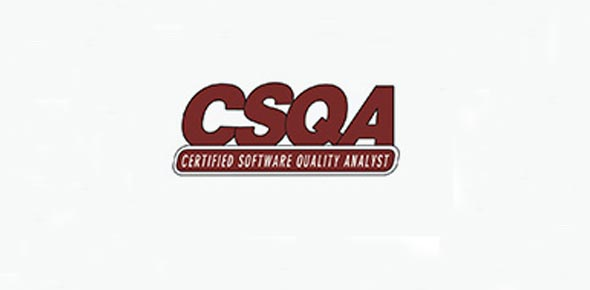# CSQA Prep - Chapter 8 - Metrics And Measurement

19 Questions | Total Attempts: 390SettingsThis quiz covers skill category 8: Metrics and Measurement

• 1.
What factors should be considered in evaluating a risk? (select all that apply) (8-26)
• A.

Profitability

• B.

Monetary Value

• C.

Schedules

• D.

Costs

• 2.
Regardless of the method used, two elements must always be considered in a risk analysis.  What are they? (8-25)
• A.

The probability of the event occurring

• B.

The impact if the event occurs

• C.

The timeline of the event

• 3.
What are some key measurements that can be used to measure a process?  (select all that apply) (8-12)
• A.

Lines of code

• B.

Products per person

• C.

Estimated vs. actual cost

• D.

Time spent fixing bugs

• E.

Deliverables completed on time

• 4.
What is ordinal data? (8-4)
• A.

Data that cannot be ranked in any meaningful order.

• B.

Data that can be ranked but differences between values are not meaningful.

• C.

Data can be ranked and can exhibit meaningful differences between values

• 5.
What are the major uses of quantitative data? (select all that apply) (8-13)
• A.

Manage and control the process

• B.

Improve the process

• C.

Manage the risks

• D.

Manage and control the product

• 6.
What are the most common ways of quantifying software size? (select all that apply) (8-9)
• A.

Lines of code

• B.

Physical space

• C.

Function points

• 7.
What are the attributes of good measurements? (select all that apply) (8-5)
• A.

Reliable

• B.

Ease of use

• C.

Valid

• D.

Timeliness

• 8.
There are inherent risks in integrating new technology.  What is the QA Analyst role in integrating new technology? (select all that apply) (8-31)
• A.

Determining the risks

• B.

Assure controls are adequate to reduce the risk

• C.

Modify existing processes for new technology

• D.

Programming the changes

• 9.
A process is defined as stable when... (8-18)
• A.

Unique situations occur

• B.

Its mean and standard deviation remain constant over time

• C.

It is always an acceptable process

• 10.
What are the distinguishing characteristics of risk? (select all that apply) (8-22)
• A.

Situational

• B.

Time-based

• C.

Interdependent

• D.

Magnitude dependent

• E.

Value-based

• 11.
Risks can be categorized as...(select all that apply) (8-21)
• A.

Technical

• B.

Programmatic

• C.

Supportability

• D.

Cost

• E.

Schedule

• 12.
What are the components that must be considered separately when determining how to manage a risk?  (Select all that apply) (8-21)
• A.

The probability that the event will occur

• B.

The event that could occur

• C.

The impact or consequence of the event if it occurs

• 13.
A _________ is a derived (calculated or composite) unit of measurement that cannot be directly observed, but is created by combining or relating two or more measures. (8-1)
• 14.
_________data has an absolute zero and meaningful ratios can be calculated. (8-3)
• 15.
The measures of central tendency are the ________, __________, and _________. (list all three) (8-3)
• 16.
The ___________ is the item at which half the items in the population are below this item and half the items are above this item.
• 17.
Which of the below represents the items which are repeated most frequently? (8-4)
• A.

Mean

• B.

Median

• C.

Mode

• 18.
This data can be ranked and can exhibit meaningful differences between values.
• A.

Ordinal data

• B.

Nominal data

• C.

Interval data

• D.

Ratio data

• 19.
Who was a strong proponent of the use of statistics that took into account common and special causes of variation?
• A.

Dr. W. Edwards Deming

• B.

Mr. Smith

Related TopicsBack to top